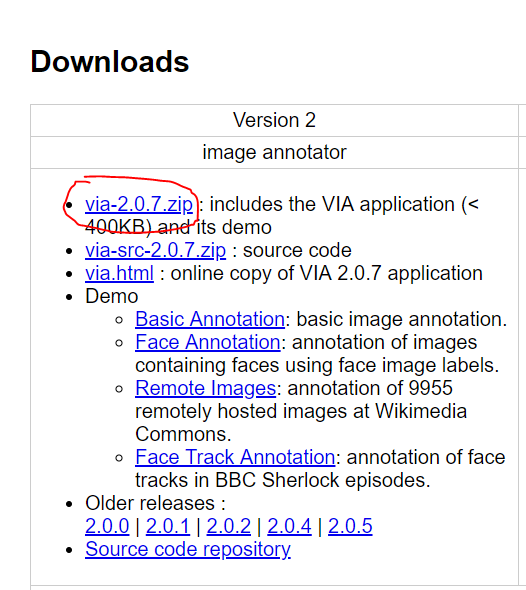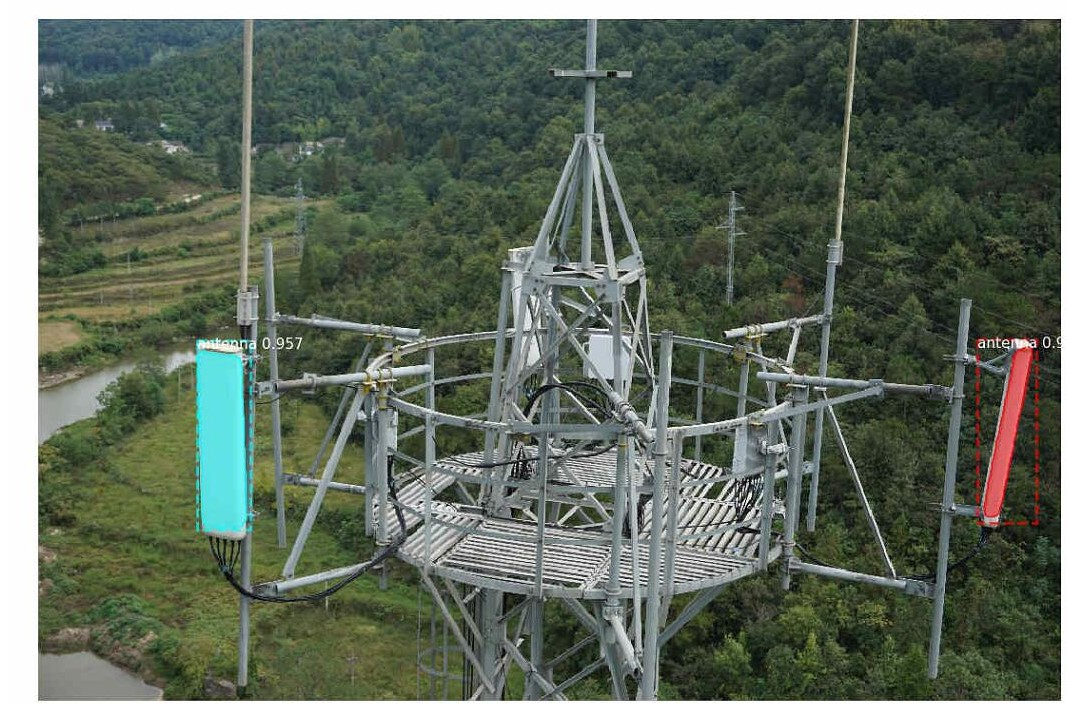本站每日ip已达10000,出租广告位,位置价格可谈,需要合作请联系站长

+关注

2019-08(82)

2019-09(116)

2019-10(8)

2019-11(8)

2019-12(12)

### 环境

1. ubuntu 16.04
2. tensorflow

### 代码前的准备

#### 1. 数据标记### 写代码中

#### 1. 看看balloon模型是怎么写的

1. 开始导了一些包，定义了一些常量
2. 定义BallonConfig，即定义了训练时的一些参数
3. 定义BalloonDataset，即定义了数据模型
4. train函数
5. 后面是一些与检测相关的函数，以及程序的命令行运行选项，都不是核心，直接略过

#### 2. 开始写

1. 首先将定义自己的config，参考BallonConfig
``````from mrcnn.config import Config
class EwattConfig(Config):
NAME = 'ewatt'
GPU_COUNT = 1
IMAGES_PER_GPU = 1
# 类型数目，本项目只需要识别一个天线，因此，为BG + antenna = 2
NUM_CLASSES = 1 + 1
# 图片的大小
IMAGE_MIN_DIM = 640
IMAGE_MAX_DIM = 1024
# 候选区域大小
RPN_ANCHOR_SCALES = (16 * 16, 32 * 128, 64 * 128, 32 * 256, 64 * 512)
# 每张图片的ROI数目
TRAIN_ROIS_PER_IMAGE = 32
# 每个epoch的迭代数目
STEPS_PER_EPOCH = 100
VALIDATION_STEPS = 20
DETECTION_MIN_CONFIDENCE = 0.9
```123456789101112131415161718```

1. 定义自己的dataset，参考BallonDataset
``````# -*- coding:utf8 -*-

from mrcnn import utils
import os
import json
import numpy as np
import skimage.draw
from skimage import io
class EwattDataset(utils.Dataset):
# 加载图片
"""Load a subset of the Balloon dataset.
dataset_dir: Root directory of the dataset.
subset: Subset to load: train or val
"""
# 添加类别，第一个参数是大类别名称，第二个参数是序号，第三个是小类别名称
# 如：
# 建议使用一个大类别名称，具体的用处，可以自己试一下

# 数据子集，不用也行，可以直接指定路径
assert subset in ["train","val"]
dataset_dir = os.path.join(dataset_dir,subset)
# 获取标记
annotations = list(annotations.values())
annotations = [a for a in annotations if a['regions']]
###############################################################
#上面这些就是从文件中加载一些数据，之前让看json文件的作用就在这儿体现，你可以每一步print看一下，在这里我就不演示了#
###############################################################
for a in annotations:
# 获取一些定义的属性（attribute）值比如region（标记区域）等，也可以获取一些自己定义的属性，并在后面做相应的修改
if type(a['regions']) is dict:
polygons = [r['shape_attributes'] for r in a['regions'].values()]
else:
polygons = [r['shape_attributes'] for r in a['regions']]

image_path = os.path.join(dataset_dir, a['filename'])
height, width = image.shape[:2]
"antenna", # 大类名称
image_id=a['filename'],  # use file name as a unique image id
path=image_path,
# 如：
# class_id = 1
width=width, height=height,
polygons=polygons)

image_info = self.image_info[image_id]
if image_info["source"] != "antenna":

info = self.image_info[image_id]
for i, p in enumerate(info['polygons']):
# 加载每一个点
rr, cc = skimage.draw.polygon(p['all_points_y'], p['all_points_x'])

# 这个函数我也不知道干啥的，修改下就完事儿了，好像是出错的时候报错的
def image_reference(self, image_id):
info = self.image_info[image_id]
if info["source"] != "antenna":
return info["path"]
else:
super(self.__class__,self).image_reference(image_id)
```123456789101112131415161718192021222324252627282930313233343536373839404142434445464748495051525354555657585960616263646566676869707172737475```
1. 修改train文件
``````# -*- coding:utf8 -*-

import os
import tensorflow as tf
import mrcnn.model as modellib
import warnings
from EwattDataset import EwattDataset
from EwattConfig import EwattConfig

# 屏蔽一些不重要的warning（强迫症必备）
warnings.filterwarnings('ignore')
os.environ['TF_CPP_MIN_LOG_LEVEL'] = '2'
tf.logging.set_verbosity(tf.logging.ERROR)

# 获取根目录
ROOT_DIR = os.getcwd()
# print(ROOT_DIR)
# 训练后模型的存储文件夹
DEFAULT_LOGS_DIR = os.path.join(ROOT_DIR, "logs")
# coco 模型

dataset_path = os.path.join(ROOT_DIR,"data")
config = EwattConfig()
def train():
# train数据
dataset_train = EwattDataset()
dataset_train.prepare()
# Val数据
dataset_val = EwattDataset()
dataset_val.prepare()
# 定义model
# 加载coco模型
"mrcnn_class_logits", "mrcnn_bbox_fc",
# 开始训练
model.train(dataset_train, dataset_val,
learning_rate=config.LEARNING_RATE,
epochs=20,
layers='all')
train()
```123456789101112131415161718192021222324252627282930313233343536373839404142434445```

#### 我的结果6 0